Thread: Finding the derivative

1. Finding the derivative

Find the derivative of the following function:

f(x) = (2x-3)^2/(x^3-7)^3

I am very confused here. Don't know what rule to use, as the exponents are outside the bracket. I would appreciate any help!

Thanks2.Originally Posted by spoc21Find the derivative of the following function:

f(x) = (2x-3)^2/(x^3-7)^3

I am very confused here. Don't know what rule to use, as the exponents are outside the bracket. I would appreciate any help!

ThanksYou will first need to use the quotient rule, and then a combination of power rule and chain rule.

Try to attempt the problem given this information and let us know where you get stuck.3.Originally Posted by spoc21Find the derivative of the following function:

f(x) = (2x-3)^2/(x^3-7)^3

I am very confused here. Don't know what rule to use, as the exponents are outside the bracket. I would appreciate any help!

ThanksUse the quotient rule. u = (2x - 3)^2 and v = (x^3 - 7)^3. To differentiate u and v, either expand and differentiate in the usual way, or use the chain rule.

4. thanks guys!

So I end up with the following result (am very unsure about it):

Derivative:

$\displaystyle 2x-3(4(x^6-32x^3+27x^2+49))$
---------------------------------
$\displaystyle (x-7)^6$

can some one please confirm if this is the correct answer.I would really appreciate it!

Thanks5.Originally Posted by spoc21thanks guys!

So I end up with the following result (am very unsure about it):

Derivative:

$\displaystyle 2x-3(4(x^6-32x^3+27x^2+49))$
---------------------------------
$\displaystyle (x-7)^6$

can some one please confirm if this is the correct answer.I would really appreciate it!

ThanksThe review process will be much easier and more effective if you post all your working (ie. show every step) rather than just giving a final answer.

6. Just in case you're interested (like many people) in avoiding the quotient rule, which can feel like overkill, and always doubles the power of the denominator, sometimes unnecessarily as here... then, how about...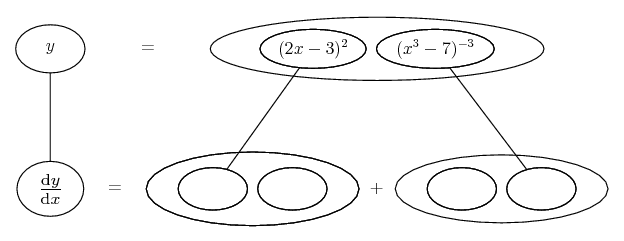... where... is the product rule, straight lines differentiating downwards with respect to x. Then you don't need u and v. You still need the chain rule, but you can zoom in on this, for example in the right hand fork...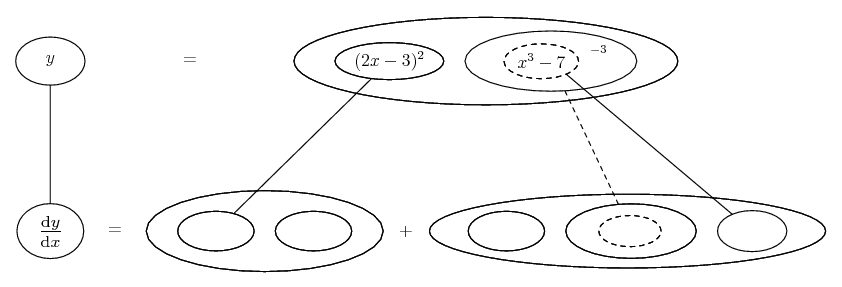... where...... is the chain rule. Straight continuous lines still differentiate downwards (integrate up) with respect to x, and the straight dashed line similarly but with respect to the dashed balloon expression (the inner function of the composite which is subject to the chain rule).

Spoiler: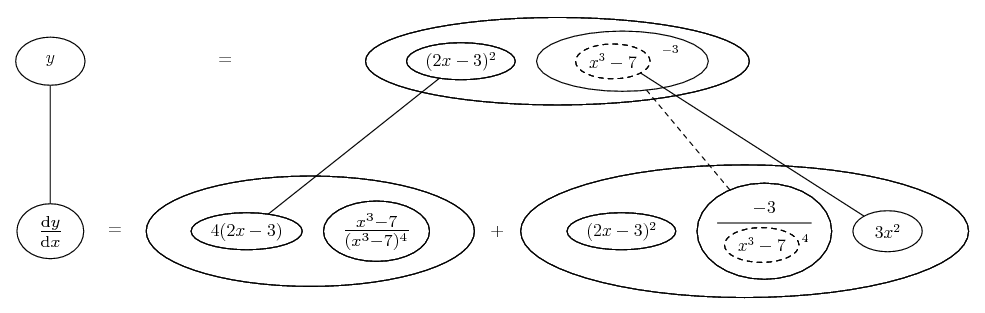However, you could zoom in on both forks...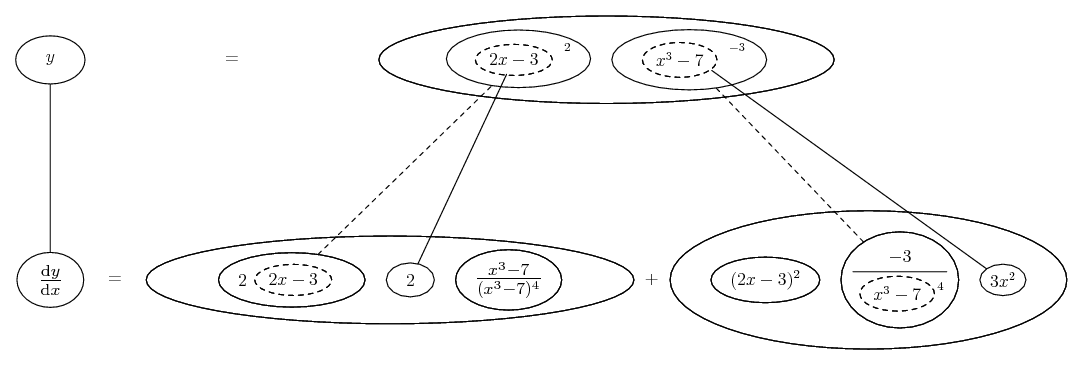... or neither...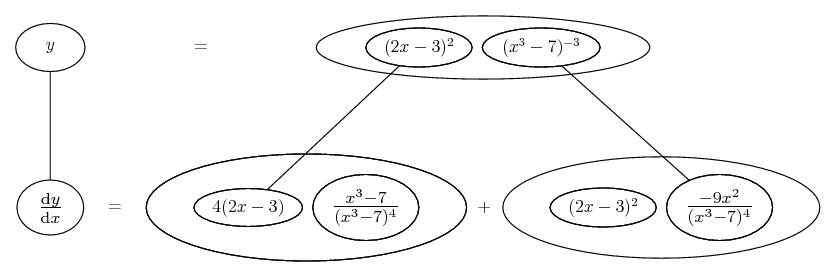... according to taste.

Another way of avoiding the quotient rule is logarithmic differentiation - taking logs of both sides of y = the function. Then solve for dy/dx in the bottom row of...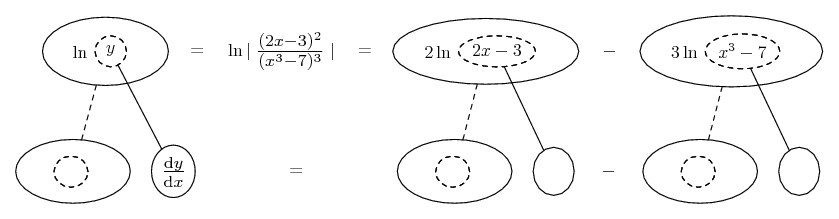Spoiler: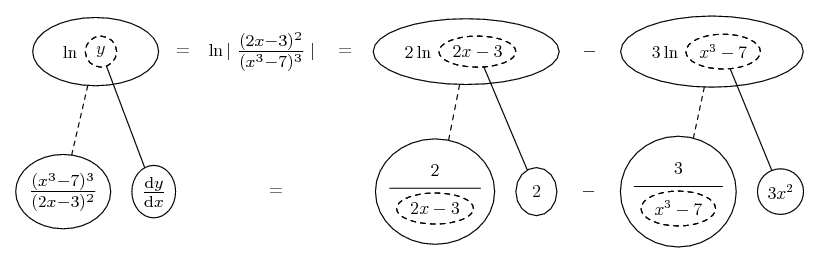_________________________________________
Don't integrate - balloontegrate!

Balloon Calculus; standard integrals, derivatives and methods

Balloon Calculus Drawing with LaTeX and Asymptote!

7. ok here's the working:

$\displaystyle f(x)=(2x-3)^2/(x^3- 7)^3$
$\displaystyle f' (x)=((x^3-7)^3 [2(2x-3)(2) ]-[3(x^3-7)^2 (3x^2 )])/[(x^3-7)^3 ]^2$
$\displaystyle f' (x)=((x^3-7)^3 [4(2x-3)]-[9x^2 (x^3-7)^2](2x-3)^2)/(x^3-7)^6$
We need to simplify:

$\displaystyle f' (x)=((x^3-7)[4(2x-3) ]-(9x^2)(2x-3)^2)/(x^3-7)^4$
We need to simplify Further:
$\displaystyle f' (x)=(2x-3)[4(x^3-7)-9x^2 (2x-3) ]/(x^3-7)^4$

thats my final answer ^^...I am supposed to represent the derivative in its simplified form..Is my working correct?

Thank you!8.Originally Posted by spoc21We need to simplify:

$\displaystyle f' (x)=((x^3-7)[4(2x-3) ]-(9x^2)(2x-3)^2)/(x^3-7)^4$
Good. (The quotient rule had to take you up to power 6 in the denominator, but you're back down again.)

We need to simplify Further:
$\displaystyle f' (x)=(2x-3)[4(x^3-7)-9x^2 (2x-3) ]/(x^3-7)^4$
Fine. Why not expand and collect in the square brackets?

Search Tags

derivative, finding